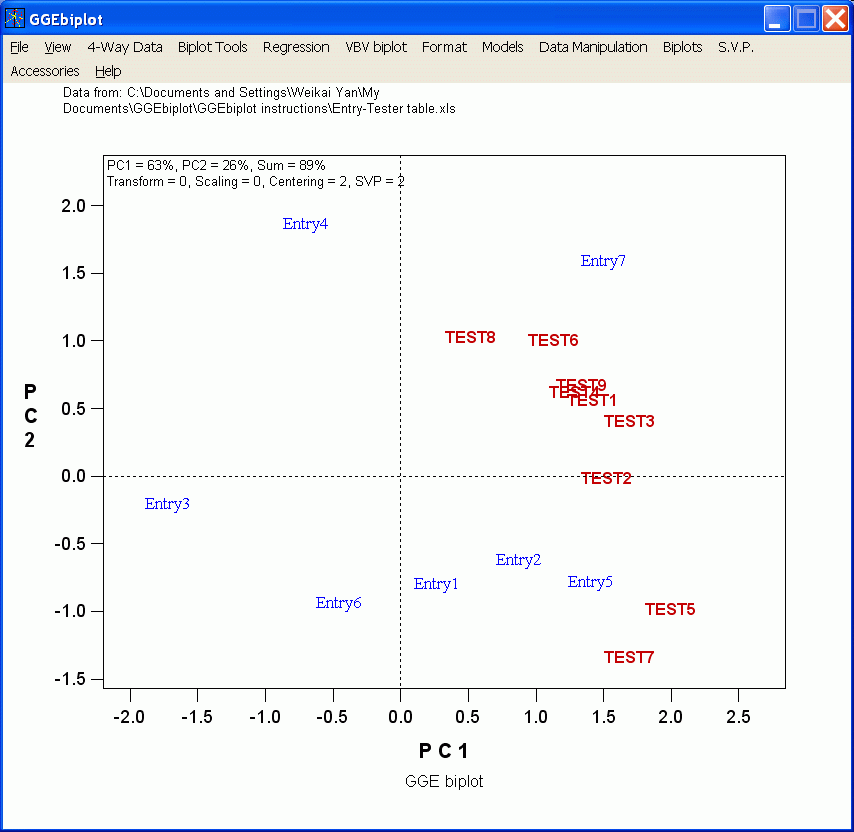Contents - Index

Four questions before trying to interpret a biplot

When a biplot is generated, the following questions must be asked before trying to interpret it:This determines what questions can be asked of the biplot and what cannot. In this biplot, the model is based on Scaling = 0 (original data not divided by anything) and Centering = 2 (tester-centered). And, the data was not transformed (Transform = 0). Therefore, this is a "GGE biplot" without scaling. It has all the interpretations of a GGE biplot

This is crucial if the purpose is to visualize the relationships among entries or that among testers. In the above biplot, SVP = 1 (entry-focused singular value partitioning). It is therefore more appropriate for entry evaluation but not so for tester evaluation. However, the choice of SVP does not affect the interpretation of entry by tester interactions.

• What is the goodness of fit

The higher the goodness of fit of the biplot, the more confident the researcher is about the interpretation based on the biplot. If only a small portion of the variation is explained, the pattern in the data  is either complicated or there is no discernible pattern at all. The above biplot explained 89% of the tester-centered data (G+GE), not of the original data.

• Are the axes drawn to scale

If the axes are not drawn to scale, the relationships among the entries and testers are distorted, which can be misleading. A biplot generated by GGEbiplot is always drawn to scale. However, watch out when reading journal papers and see if a biplot is stretched to fit the space. If so, the biplot may be misleading.

All these pieces of information are explicitly indicated on the top-left corner of the biplot:

1) Goodness of fit: PC1= 63%, PC2 = 26%, Total = 89%;

2) Data transformation method: Transformation = 0;

3) Data Scaling method: Scaling = 0;

4) Data Centering method: Centering = 2;

5) Singular value partitioning: SVP = 1; and

6) Drawn to scale: It is drawn to scale, which is always true in biplots generated by GGEbiplot.